##1. Steiner ellipses

One of the simplest methods to define the two Steiner ellipses of a triangle is to use a homography F, that maps the vertices of an equilateral triangle t = (ABC) onto the vertices of an arbitrary triangle t' = (A'B'C') and the center D of t onto the centroid D' of t'. These conditions uniquely determine F (up to permutation of vertices). Let a, b be the circumcircle and incircle, correspondingly of t. Their images a' = F(a) and b' = F(b) are correspondingly the exterior and interior Steiner ellipses of t'.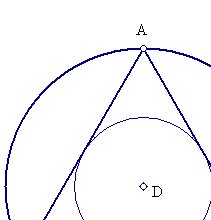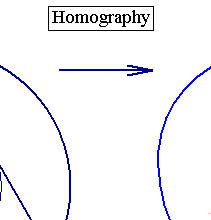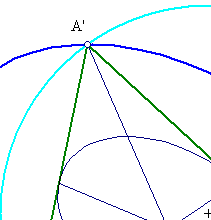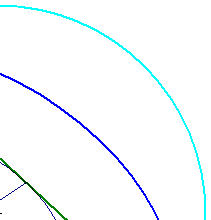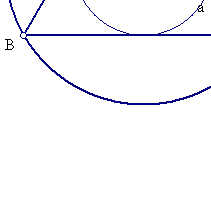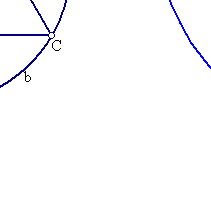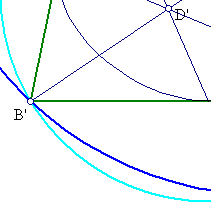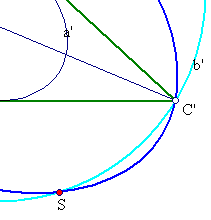From the general properties of homographies result the main properties of Steiner's ellipses of the triangle t':
1) From the invariance of cross-ratio and the properties of D, D' follows that the medians of t are mapped to the medians of t'.
2) This implies that the line at infinity is mapped to the line at infinity, hence the homography is an affinity.
3) Affinities, respect parallelity, length-ratios, area-ratios and map circles to ellipses.
4) This implies that the inner circle is mapped to the inner ellipse, that touches the sides of t' at their middles.
5) From (3) follows also that the tangent to the outer ellipse at the vertex is parallel to the opposite side of the triangle.
6) The centers of the two ellipses coincide with D' and the ellipses are homothetic with ratio 2 w.r. to that point.
7) The inner ellipse is the one inscribed with the maximum area, and the outer is the one circumscribed with the minimum area.
8) Since the affinity preserves area-ratios, it follows that
area(inner Steiner) = (pi/(3*sqrt(3)))*area(A'B'C') and
area(outer Steiner) = (4*pi/(3*sqrt(3)))area(A'B'C').

##2. Other means of construction

Another way to construct these ellipses results from properties of equilateral transferred by the homography F to the general configuration.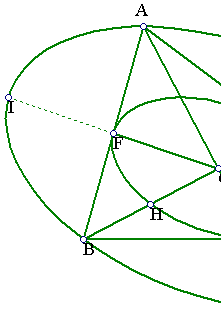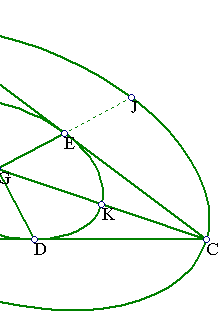In fact, the ellipses of Steiner and the sides/vertices of the triangle intercept on the medians of the triangle equal segments. Thus, BH = HG = GE = EJ, CK = KG = GF = FI etc.
Thus, the conics can be constructed from five easy to find points through which pass (using the tool constructing a conic which passes through five points in general position).

##3. The Steiner point

The Steiner point of a triangle is the fourth intersection point of the circumcircle with the outer Steiner ellipse. An easy construction of this point is given in SteinerPoint.html . In the Kimberling's catalog of triangle centers (http://faculty.evansville.edu/ck6/encyclopedia/ETC.html) it has the notation X(99).